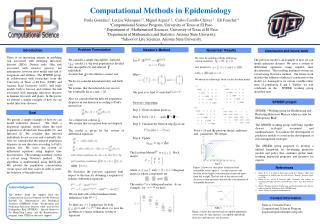DownloadDownload PresentationReferences

# References

Télécharger la présentation## References

- - - - - - - - - - - - - - - - - - - - - - - - - - - E N D - - - - - - - - - - - - - - - - - - - - - - - - - - -
##### Presentation Transcript

1. Problem Formulation SPIDER project References Contact Information Computational Methods in Epidemiology Paula Gonzalez1, Leticia Velazquez1,2, Miguel Argaez1,2,Carlos Castillo-Chávez 3, Eli Fenichel4 1Computational Science Program, University of Texas at El Paso. 2Department of Mathematical Sciences, University of Texas at El Paso. 3Department of Mathematics and Statistics, Arizona State University. 4School of Life Sciences, Arizona State University. 4. Newton’s Method Introduction Numerical Results Conclusion and future work There is an increasing interest in modeling risk associated with emerging infectious diseases (EIDs). Disease risks, (like risk associated with invasive species), are endogenous, however most model treat risk as exogenous and intrinsic. The SPIDER group, in collaboration with researchers from the University of Texas at El Paso (UTEP) and Arizona State University (ASU), will study models both to forecast and estimate the risk associated with emerging infectious diseases in humans livestock and plants. In this poster we present a simple example of how we can model infectious diseases.. We solve the problem with the followings initials conditions and parameters with We obtain the followings blocks in the Jacobian matrix The previous model is an example of how we can model infectious diseases. We solve a system of differential equations using implicit time discretization. The resulting nonlinear system are solved using Newton’s method. Our future work includes the addition of physical constraints to the model, i.e, nonnegative on certain variables likes rates of population S and I. Further, we will colaborate in the SPIDER working group described next. We consider a simple Susceptible - Infected ( ) model, i.e. the total population is divided into susceptible (S) and infected (I) individuals. Assume that is the effective contact rate. We do not consider natural mortality and birth rate. We assume that the infected do not recover, but eventually die at a rate . Also we consider that the infected population disperses in one direction according to Fick's motion law: for a dispersion constant . We assume that susceptible does not disperse. The model is given by the system of differential equations Let Where Our goal is to find such that . Newton's Algorithm: Step 1: Given an initial point . Step 2: For h = 0, 1, … until . Step 3: Calculate the Newton step from: Step 4: Update . • SPIDER: “Working group for Synthesizing and Predicting Infectious Disease while account for Endogenous Risk”. • The SPIDER working group will bring together disease ecologist, economist and mathematicians. To facilitate the development of predictive models to assist in the development of risk management strategies. • The SPIDER group proposed to develop a unified framework for developing predictive models and policy that combines global trade, learning, inspection programs, and incentive for experts. Goal We present a simple example of how we can model infectious diseases. We study a dispersion epidemic model where the total population is divided into Susceptible (S) and Infected (I). We consider that infected individuals do not recover and eventually die. Also we consider that the infected population disperses in one direction according to Fick’s motion law. We solve the system of differential equations using implicit time discretization. The resulting nonlinear system is solved using Newton’s method. The algorithm is implemented using MATLAB. We plotted the population concentration versus space and time scales in order to study the behavior of the individuals. For n = 20 and the previous initial conditions and parameters. We obtain The Jacobian Matrix is a block matrix: where are diagonal matrices whose components are The matrix C is a tridiagonal matrix. As an example , for we have Figure 1 Figure 1 shows six time plots of infected and susceptible versus space x . As time increases the location of the largest concentration of infected move from left to right. The left side of the infected will decrease as time increases because the concentration of susceptible decreases. We discretizethe previous equations with respect to the time for obtaining a sequence of ordinary differential equations Horan, R. D., C. A. Wolf, E. P. Fenichel, and K. H. J. Mathews. 2008. Joint management of wildlife and livestock disease. Environ. Resource Econ. 41:47-70. Horan, R. D., and E. P. Fenichel. 2007. Economics and ecology of managing emerging infectious animal diseases. Am. J. Agr. Econ 89:1232-1238. Project: Working group for Synthesizing and Predicting Infectious Disease while account for Endogenous Risk. SPIDER group. White, Robert. Computational Mathematics. Acknowledgments The authors thank the support from the Computational Science Program and the National Institute for Mathematical and Biological Synthesis (NIMBioS) Grant, "Synthesizing and Predicting Infectious Disease while account for Endogenous Risk (SPIDER)". Special thanks to Dr. Ming-Ying Leung and the Bioinformatics program from UTEP for the travel support. We use half cells at the boundaries finite differences with . So there are unknowns for both and , this allow s to pose the problem as a square nonlinear system of equations. Paula A. Gonzalez-Parra Computational Sciences Program pagonzalezparra@miners.utep.edu Figure 2 Figure 2 shows the infected and susceptible population versus time. As time increase, susceptible individuals decreases and infected one increases.